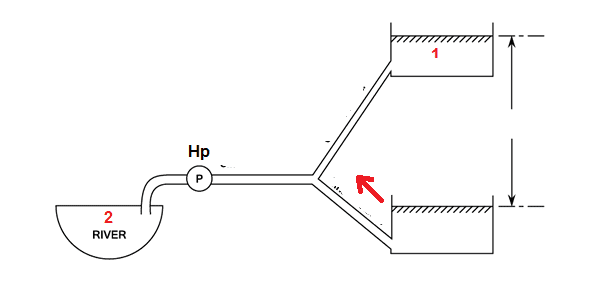# Can I apply Bernoulli's equation to this situation?

Atouk
TL;DR Summary
Necessary conditions for Bernoulli's theorem.Can Bernoulli's equation be applied between points 1 and 2, ignoring the another tank ?

Last edited:

Mentor
Summary:: Necessary conditions for Bernoulli's theorem

https://www.physicsforums.com/attachments/263163
Can Bernoulli's equation be applied between points 1 and 2, ignoring the another tank ?
What do you think?

Atouk
I would say "of course not". But
What do you think?
I would say "of course not", but I can not explain what happens with the steamline between 1 and 2...

Mentor
All the water would flow from the upper reservoir to the lower reservoir on the right. There would be no flow from 2 to 1 unless a huge flow were forced by the pump. It would have to provide a pressure of at least 10 psi.

Atouk
All the water would flow from the upper reservoir to the lower reservoir on the right. There would be no flow from 2 to 1 unless a huge flow were forced by the pump. It would have to provide a pressure of at least 10 psi.
I see, but supposing that I have all the information about the points 1 and 2
(speed, pressure and height), could I be able to apply Bernoulli's equation and figure out Hp?
My question is conceptual, the values don't matter.

Last edited:
Mentor
I see, but supposing that I have all the information about the points 1 and 2
(speed, pressure and height), could I be able to apply Bernoulli's equation and figure out Hp?
My question is conceptual, the values don't matter.
I think that if you specify the flow at the pump, you can determine the pressure at the pump and the flows in the two arms using Bernoulli.

Atouk
I think that if you specify the flow at the pump, you can determine the pressure at the pump and the flows in the two arms using Bernoulli.
So, in an ideal situation (steady flow, without losses in pipes, etc.)
p1 / ρ + (v1^2) / 2 + g*h1 = p2 / ρ + (v2^2) / 2 + g*h2 - Hp ?

Mentor
So, in an ideal situation (steady flow, without losses in pipes, etc.)
p1 / ρ + (v1^2) / 2 + g*h1 = p2 / ρ + (v2^2) / 2 + g*h2 - Hp ?
To get your feet wet, start out by considering the problem where there is no flow from the pump.

Homework Helper
Gold Member
What does the red arrow mean?

All the water would flow from the upper reservoir to the lower reservoir on the right. There would be no flow from 2 to 1 unless a huge flow were forced by the pump. It would have to provide a pressure of at least 10 psi.
Where on Earth are you getting 10 psi from here?

Summary:: Necessary conditions for Bernoulli's theorem.

View attachment 263167
Can Bernoulli's equation be applied between points 1 and 2, ignoring the another tank ?

In general, this does not look like a situation where Bernoulli would apply because based on the diagram alone, I strongly suspect that there are significant viscous losses here, especially given that 2 is labeled as a river with what appears to be a free surface well below the surface of 1. Bernoulli applies to situations without significant viscous loss, and I don't see how that can be the case here.

Mentor
Where on Earth are you getting 10 psi from here?
If I remember correctly, the OP showed some dimensions on his original post (which was later edited).

Mentor
In general, this does not look like a situation where Bernoulli would apply because based on the diagram alone, I strongly suspect that there are significant viscous losses here, especially given that 2 is labeled as a river with what appears to be a free surface well below the surface of 1. Bernoulli applies to situations without significant viscous loss, and I don't see how that can be the case here.
After further consideration of this situation, I totally agree.

If I remember correctly, the OP showed some dimensions on his original post (which was later edited).
Ah, that would explain it. I first saw it with no dimensions, so your claim seemed totally arbitrary.